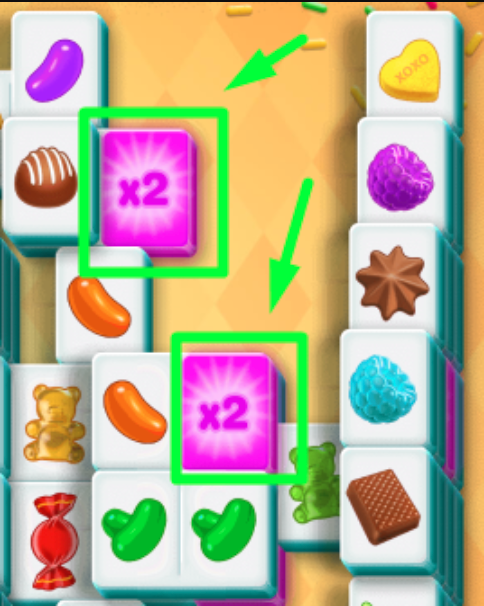• Points per match = 200 points

• Tile-Matching Multiplier Bonus:

This multiplier equals the number of tiles you've matched in a row: X2, X4, X6 (no more than X12)• Multi-Match Bonus:
• Multi-matches occur when you make a match with the same symbols as the previous match
• The first multi-match will display X2, then X3, and so on.
• Multi match bonus points = 500 * multi-match multiplier points
• Time Bonus = Number of seconds left * 400
• Shuffle = Reduces the number of seconds left by 20 seconds

Final score = (all match points) * multiplier + multi-match bonus + time bonus